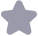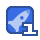### C语言二级考试真题收藏

(11)下列不合法的用户标识符是() A)j2 KEY B) Double C)4d D)8 (12)下列不合法的数值常量是()。 A)011 B) lel C)8.0E0.5 D) Oxabcd (13)下列不合法的字符常量是()。 A)"018" B)"" C)'N D) (14)表达式3.6-5/2+1.2+5%2的值是()。 A)4.3 B)4.8 C)3.3 D)3.8 (15)下列能止确定义字符串的语句是() A) char str[]=1064, B) char str-Kx43 C) char str= D)char str[]=0 (16)下列数组定义中错误的是()。 A)intx[I={0} B)intx[23}{{1,2},{34},{5,6}; C)intx[I[3}={1,2,3},{4,5,6}; D)intx[2I3}{1,2,34,5,6} (17)若要求从键盘读入含有空格宇符的字符串,应使用函数() A) getc() B)gets( C)getchar() D) scanf( (18)下列4个程序中,完仝正确的是()。 A)#include <stdio. h> B)#include <stdio .h> InaIn( i/programming*/ 1//programming/* printf("pi printf("programming! n);1 C) #include <stdio. h> D) include <stdio. h> main() nain( / programming ,programming printf("programming! n): 1 printf("programming! in: J (19)若有定义: float X=1.5;inta=1,b=3,c=2;则正确的 switch语句是()。 A) switch(x) B)switch(int)x) i case 1.0: printf("\ n); icase I: printf("*n") case 2.0: printf("**\n); 5 case 2: printf("*n); C)switch(a+b) D)switch (a+b) i case I: printf("*n"); { case I:pint("*Ⅶn"); case 2+1: printf("**n )33 case cprint tt**mn");} (20)若各选项中所用变量已正确定义,funO函数中通过rum语句返回一个函数值,下列 选项中错误的程序是()。 A) main() B) float fun(int a, int b)f.,.j {……X=fun(2,10);… main() float fun (int a, int b)i..3 {…X=fun(ij);……} 级C语言程序设计试卷第2页(共12页) C)float fun(int, int) D)main( main() float fun (int I, int j {…X=fumn(2,10);……} ……X=un(ij); float fun( int a int b){…} b){…} (21)在下列给出的衣达式中,与 while(E)中的(E)不等价的衣达式是()。 A)(!E B)(E>0E<0) C)(E==0) D)(E!=0) (2)要求通过 while循坏不断读入字符,当读入宇母N时结束循坏。若变量己正确定义, 下列正确的程序段是() A) while((ch=getchar()!=N)printf("%c", ch) B) while(chgetchar()!=N,) printf("%oc",ch) c) whilc(ch=getchar(==,)printf("%c",ch) D) while((ch=getchar())==N )printf("%" ch); (23)已定义下列函数: int fun(int*p) relum fun函数返回值是()。 A)不桷定的值 B)·个整数 C)形参p中存放的值 D)形参p的地址值 (24)若有说明语句: double*p,a;则能通过 scant语句正确给输入项读入数据的程序段是 A)*p&a; scanf(%1f",p) B)*p&a: scanf("%f",p) C)p=&a; scanf(%1f" *p); D)p=&a; scanf("%1f,,p) (25)现有以下结构体说明和变量定义,如图所示,指针p、q、【分别指向一个链表中连续 的3个结点。 data next data next ata next B p struct node i har data; truct node *next;p, a, r 现要将q和r所指结点交换前后位置,冋时要保持链表的连续,下列不能完成此操作 的语句是() A)q >next=r->next; p->next=r, r->next=q B)p->next=r; q- >next=r->next; T->next=q C) q >next=r->next; r->next=q: p->next=r; D)r->next=g: p->next=r; q->next=r->next (26)有下列程序段 int x; int y inta[]={2},b[]={3,4}; struct st c=(10, a, 20, b 级C语言程序设计试卷第3页(共12页) pt=c 下列选项中表达式的值为11的是()。 A) B) pU D)(pt+)->x (27)设垆为指向某二进制文件的指针,且已读到此文件末尾,则函数feof(fi)的返回值为 A) EOF B)非0值 C)0 D) NULL (28)设有以下语句 int a=lb= c=a(b<<2) 执行后,C的值为 A)6 B)7 C)8 D)9 (29)有下列程序 Include <stdio.h> main() i char cl, c2, c3, c4, c5, c6 scanf("%c%c%c%oc", &cl, &c2, &c3, &c4) c5=getchar(); c6=getchar() putchar(c1): putchar(c2) printf("%c%", c5, c6) 程序运行后,若从键盘输入(从第1列开始) 123<CR> 45678<CR 则输出结果是()。 A)1267 B)1256 C)1278 D)1245 (30)有下列程序 i int y=10 while(y--); printf("y=%od\n"y) 程序执行后的输出结果是() B)y=-1 C)y=1 D) while构成无限循环 (31)有下列程序: main( {inta=0,b=0.=0,d=0 f(a-1)b=1;c=2 else printf(""od, %od, %/od, %odn, a, b, c, d) 级C语言程序设计试卷第4页(共12页) 程序输出()。 A)0,1,2,0 B)0.0,0,3 C)1,l,2,0 D)编译有错 (32)有下列程序 main() for(i=0;1<2;计++) x++; for-0j<-3j++) i if(i%2)continue printf(x=od\n,x) 程序执行后的输出结果是() A)x=4 B)x-8 C)x=6 D)x=12 (33)有下列程序 int funl(double a return a*=a; 3 int fun(double x, double y) i double a=0, b=0; afunl(); b=fun1(y) return (int (a+b); Inain( { double w;w-fun2(1.1,2,0;……} 程序执行后变量w中的值是() A)5.21 B)5 C)5.0 D)0.0 (34)有下列程序 main( {inti,t][3}={9,8,7,6,5,4,3,2,1}; for(i=0; 1<3; 1++) printf("%d", t[2-1D) 程序执行后的输出结果是() A)753 B)357 C)369 D)751 (35)有下列程序 fun(char p[l[10j) i int n=0, 1; for(i=0;i<7;i++) if(p[[o]= return n; 级C语言程序设计试卷第5页(共12页) maIr i char str[ =f Mon", Tue","Wed", Thu", Fri, Sat","Sun") printf("d\n", fun(str)); 程序执行后的输出结果是()。 A)1 B)2 C)3 D)0 (36)有下列程序 {inti,s=0,t]={,2,3,4,5,6,7,8,9}; for(i=0;<9;i+=2)s+=*(t+i); printf(%d\n", S) 程序执行后的输出结果是 A)45 B)20 C)25 D)36 (37)有下列程序: void funI(char p) har hile (q!0 {(*q)++;q++;} main( i char al =f Program",'p p&a3]: funI(p): printf("%sn", a) 程序执行后的输出结果是()。 A) Prohsb B) Prphsbr C) Progsbn D) Program (38)有下列程序 void swap(char x, char y i char t main() i char *sl=abo wap(sl, 2); printf("%S, %sn, s1, S2) 程序执行后的输出结果是( A) 123. abc B) abc 123 C) lbc. a23 D)321,cb 39)有下列程序: 级C语言程序设计试卷第6页(共12页) int fun(int n) d if(n==lreturn I; return(n+fun(n-1); main( anrC%d, &x); xfun(x): printr"%d\n", x) 执行程序时,给变量ⅹ输入10,程序的输岀结果是()。 A)55 B)54 C)65 D)45 (40)有下列程序: fun(int x[l, int n) i static int sum=0, i for(i=0 i<n; i++) sum+=x[i] rcturn sut main {inta[={1,2,3.4,5},b]={6,7,8,9},s=0 slun(a, 5)+lun(b, 4); printr(%odn", S); 程序执行后的输出结果是( A)45 B)50 C)60 D)55 (41)有下列程序: main() union i char ch int d 21: printf( %ox, %xn", sch[0l, sch[ID) 在16位编译系统上,程序执行后的输出结果是()。 A)21,43 B)43,21 C)43.00 D)21,00 (42)有下列程序: main( {char本p[]={"3697","2584"} int i, j;long num=0; for(i=0;i<2;i++) while(pill! =0) 级C语言程序设计试卷第7页(共12页) i(i]-0)%2)num=10nump[i][j]-"0 printf( ood\n", num 程序执行后的输出结果是() A)35 B)37 C)39 D)3975 (43)执行下列程序后, test. txt文件的内容是(若文件能正常打开)() #include <stdio. h> main( I FILE fp char *s1=Fortran,*s2-"Basic" if((fp=fopen("test. txt,wb))==NULL) iprinlr"Can't open esL Lxt lile n ) exit(1): 1 fwrt(s1,7,1,fp);/把从地址sl开始的7个字符写到fp所指文件中* ek(fp,OL, SEEK SET),/*文件位置指针移到文件开头* write(s2, 5, 1, fp); fclose(fp) A) Basican B) Basicfortran C) Basic D) Fortran Basic (44)下列叙述中错误的是()。 A〕C语言源程序经编译后生成后级为obj的目标程序 B)￠程序经过编译、连接步骤之后才能形成一个真正可执行的二进制机器指令文件 C)用C语言编写的程序称为源程序,它以 ASCII代码形式存放在一个文本文件中 D)C语言中的每条可执行语句和非执行语句最终都将被转换成二进制的机器指令 (45)下列叙述中错误的是()。 A)算法正确的程序最终一定会结束 B)算法止确的程序可以有零个输出 C)算法正确的程序可以有零个输入 D)算法正确的程序对于相同的输入一定有相同的结果 (46)下列叙述中错误的是() A)C程厅必须由一个或一个以上的函数组成 B)函数调用可以作为个独立的语句存在 C)若函数有返回值,必须通过 return语句返回 D)函数形参的值也可以传回给对应的实参 (47)设有下列定义和语句 char str="Program", "p 则下列叙述中正确的是() A)岑p与st的值相等 B)sr与p的类型完全相同 级C语言程序设计试卷第8页(共12页) C)sr数组长度和p所指向的字符串长度相等 D)数组str中存放的内容和指针变量p中存放的内容相同 (48)下列叙述中错误的是( A)C程序中的# include和# define行均不是C语句 B)除逗号运算符外,赋值运算符的优先级最低 C)C程序中,j+;是赋值语句 D)C程序中,+、-、*、/、%号是算术运算符,可用于整型和实型数的运算 (49)下列叙述中正确的是()。 A)预处理命令行必须位于C源程序的起始位置 B)在C语言中,预处理命令行都以"#"开头 C)每个C程序必须在开头包含预处理命令行:# includesstdio.h> D)C语言的预处理不能实现宏定义和条件编译的功能 (50)下列叙述中错误的是()。 A)可以通过 typedef增加新的类型 B)可以用 typedef将已存在的类型用一个新的名字米代表 C)用 Typedef定义新的类型名后,原有类型名仍有效 D)用 typed可以为各种类型起别名,但不能为变量起别名 填空题(每空2分,共40分) (1)对长度为10的线性表进行冒泡排序,最坏情况下需要比较的次数为【1】 (2)在面问对象方法中,【2】描述的是具有相似属性与操作的一组对象 (3)在关系模型中,把数据看成是二维表,每一个二堆表称为一个【3】 (4)程序测试分为静态分析和动态测试。其中【4】是指不执行程序,而只是对程序文 本进行检查,通过阅读和讨论,分析和发现程序中的错误 (5)数据独立性分为逻辑独立性与物理独立性。当数据的存储结构改变时,其逻辑结构可 以不变,因此,基于逻辑结构的应用程序不必修改,称为【5】 (6)若变量a,b已定义为int类型并赋值21和55,要求用 printf函数以a-21,b-55的形 式输出,请写出完整的的输出语句【6】。 (7)下列程序用于判断a、b、c能否构成三角形,若能,输出YES,否则输出NO。当给a、 b、c输入三角形三条边长时,确定a、b、c能构成三角形的条件是需同时满足三个条 件:a+b>c,a-c>b,b+c>a。请填空 main( float a b scanf(%f%f%f", &a, &b, &c) f(【】) printf("YFSn"):*a、b、c能构成三角形* else printf("NOn");*a、b、c不能构成三角形* (8)下列程序的输出结果是【8】。 main( {inta={{1,2,9},{3.4,8},{5,6,7}},i,s=0; for(i=0;1<3;计++)s+=a[ii+a[i[3-1-1]; printf("%d\n" s) (9)当运行下列程序时,输入abcd,程序的输出结果是:_【9】。 级C语言程序设计试卷第9页(共12页)

...展开详情

2326655C语言二级考试真题 33积分/C币 立即下载
1/1333积分/C币 立即下载 ＞